# Types and functions

Video
• 16 minutes

We’re going to begin by talking a little bit about the grammar, or the essential structure, of the Haskell language. This means starting with a notion of what types and functions are and thinking about the relationship between the two.

## Types

We can think of types as being like sets. When we think about numbers, for example – “numbers” is not itself exactly a set. There are different sets of numbers that are useful for different things. For example:

• The integers, which include negative and positive whole numbers.
• The “natural” numbers,Some people like to include zero among the rationals, others prefer not to. The `Natural` type in Haskell does include zero. which include just the positive whole numbers.
• The “rational” numbers, which includes all the whole numbers like integers, but also all the fractional numbers.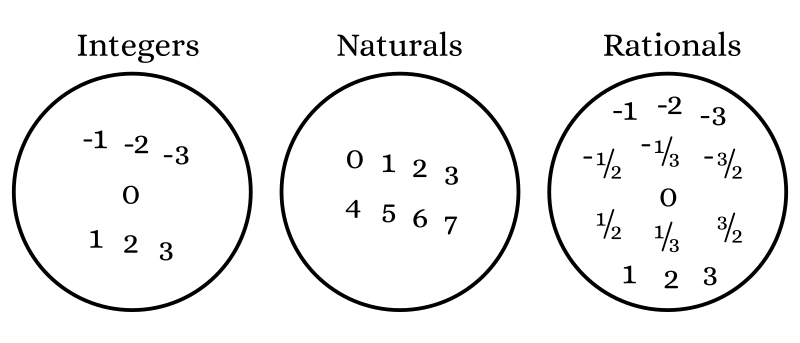The rationals represent a broader notion of numbers than the integers, which are in turn a larger idea than the naturals. Even the set of rationals does not fully encompass everything that we call “numbers” – there are still more sets of numbers, and more ways to describe things like counting and measurement using different sets depending on what we want to include and what purpose we have.

In general, what we call “numbers” are not a fixed set of things, but a group of sets that have some things in common. For example, we can do addition with members of any of these sets – we can add integers, we can add natural numbers, and we can add rational numbers. We can subtract and multiply within all of these sets. So in some sense, we know what a number is not because it is included in some specific set of all numbers, but because of what we can do with a set of numbers.

## Classes

In Haskell, the concept of “being a number” is represented through a typeclass. We have types in Haskell which are like sets, and we have classes – which is sort of a larger concept – that defines some operations that multiple sets have in common.

We have a typeclass called `Num`, and it includes a bunch of types of numbers, including `Integer`, `Natural`, `Rational`, and some other types of numbers. And it gives operations that can be performed on them, such as addition, multiplication, and subtraction.

That is how we represent this concept of a number. We have sets that include actual values like 1, 2, and 3. Those are sets like `Integer` and `Natural`, and `Rational`, and we call them types. Then we have a typeclass that tells us what all of these types have in common, the operations we can do with them that they all share.

## More types and classes

There are types that aren’t numeric, of course, because there’s lots of types of data that we have to deal with. There’s one set we have that has only two values in it, called `Bool` – there’s only `False` and `True` in that set. We have another type called `Char` that includes alphabetic characters, anything you can type with a standard keyboard, and whole bunch of other stuff.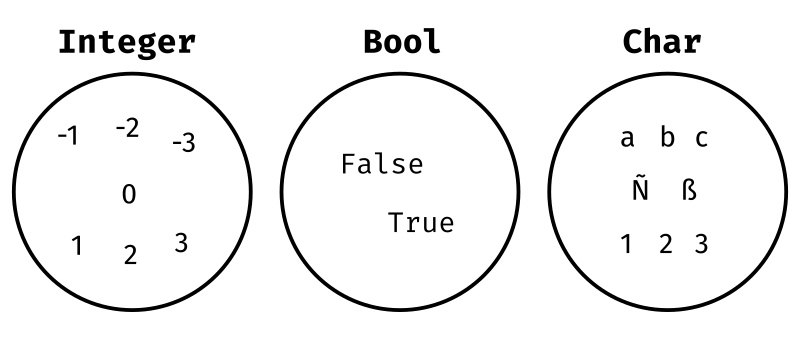`Integer`, `Natural`, and `Rational` are all conceptually similiar enough that it’s easy enough to see that we can do things with addition with all of them. But can we think of a typeclass, maybe, that could include both character types and numbers? What could a set of characters and a set of numbers have in common?

For one thing, they can be put into an order. There’s a typeclass for that, called `Ord`. That’s where the comparison operations like greater-than (`>`) and less-than (`<`) are. One value is less than another (`x < y`) if `x` comes before `y` in the ordering.

All of the types we’ve mentioned so far can also be tested for equality. Does `'a' == 'a'`? Yes. Does `'a' == 'b'`? No, these are different characters. Likewise `1 == 1` is true, and `1 == 2` is false. Our typeclass for this in Haskell is called `Eq`, short for equality, and it defines this `==` operator.

## Functions

Functions are mappings between sets.

You might have seen when you were in school, worksheets like this.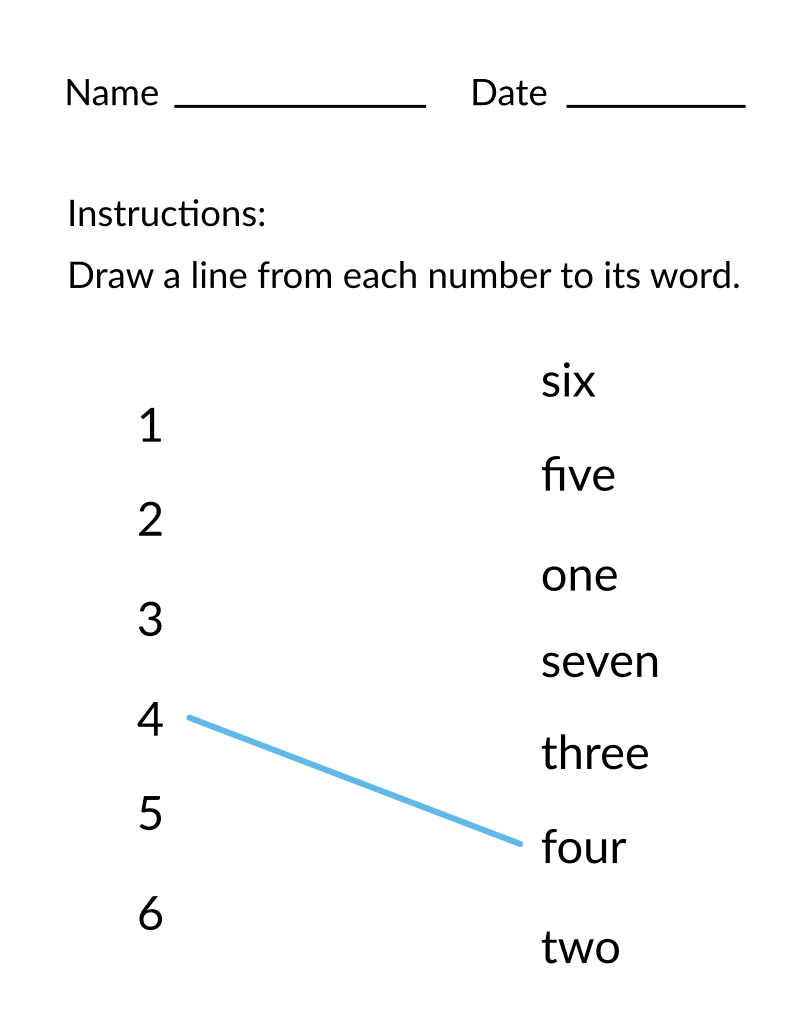On the left we have numerals, on the right we have the words for numbers, and we are asked to draw a line from each numeral to how it’s spelled. In our elementary schools we had to do a lot of these kinds of exercises.

This is our mental picture of what defining a function is like: drawing lines from the things on the left to the things on the right, based on some rule that tells us what makes the matchings meaningful. A function is a description of how an element from one set gets matched toIt may happen to be that the input and output sets are the same set; this is okay. an element in another set.

Keep this notion in mind – a function is the process by which we match up the column on the left to the column on the right – while we think about how to express this function in Haskell. Our goal will be to match up numbers to their English spelling.

We’ll call this function “spell”. We’ll first write the type declaration for the `spell` function.

``spell :: Integer -> String``

You can read the double-colon (`::`) as “has the type” – so what we have written here is “`spell` has the type `Integer -> String`”.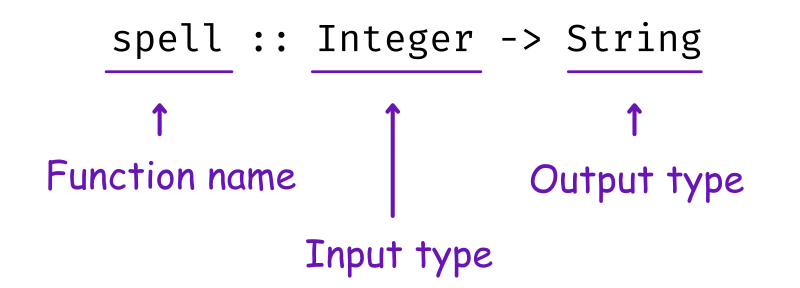So the type of the inputs to this function are integers (1, 2, …), and the outputs are strings (“one”, “two”, …).

That was the type declaration; now let’s add the function definition.

``````spell :: Integer -> String

spell int =
case int of
1 -> "one"
2 -> "two"
3 -> "three"
4 -> "four"``````

The first thing we write is `spell` again – the name in the definition has to be the same as the name in the type declaration. The next thing we see, `int`, is a variable – we call is the parameter to this function. That means that when we apply `spell` to some nunber, that number is going to take the place of this variable that we have named `int`.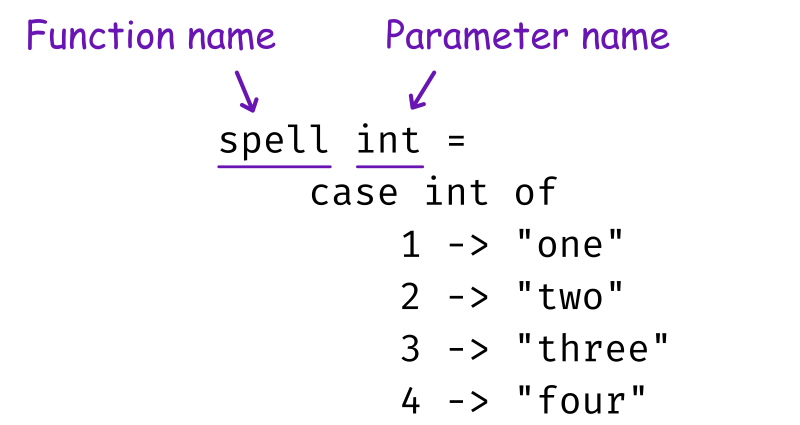On the left of the equals sign is where we have bound this variable name, and on the right of the equals sign is where we are using this variable.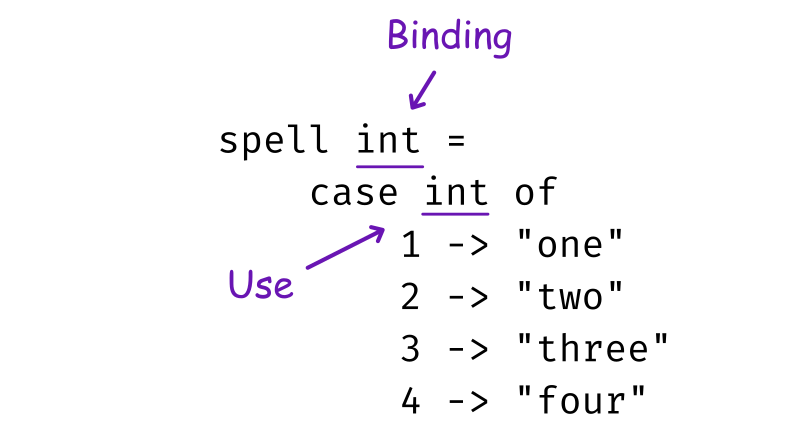After `case int of`, each line contains an arrow that matches a number on the left to a word on the right. Notice that the output strings are in quotation marks, which is how we write strings in Haskell. The numbers are written plainly without any extra adornment.

When we apply `spell` to some numeral, the evaluator will look down the list to see which one of these cases on the left it matches, and then return the corresponding output string on the right.

Enter the type declaration and function definition into your `main.hs` file, and go to the REPL to try applying this function to some arguments.

``````λ> :load main.hs
[1 of 1] Compiling Main    ( main.hs, interpreted )

We need to apply the `spell` function to some argument; pick a number 1 through 4.

``````λ> spell 2
"two"``````

Now, it would be quite impossible to write this particular function to list every possible integer. So this is what we call a partial function: it doesn’t map every possible value from the input set to an output. When we wrote the type `Integer -> String`, we were asserting that for any `Integer`, you can get a `String`. But this isn’t really true – We haven’t written a function for all of the integers, we’ve written a function for just 1, 2, 3, and 4. For some integers, this function will simply fail. So this is a partial function; it only covers part of the input set.

``````λ> spell 122
*** Exception: main.hs:(4,5)-(8,19): Non-exhaustive patterns in case``````

There’s a couple of things we could do to improve this. One thing we might do is add a catch-all case as the end. We use an underscore as a kind of wildcard, signifying “anything other than 1, 2, 3, and 4”. In this situation, we’re going to return the string “I don’t know this number!”

``````spell :: Integer -> String

spell int =
case int of
1 -> "one"
2 -> "two"
3 -> "three"
4 -> "four"
_ -> "I don't know this number!"``````

Now, we can go to our REPL and reload, and we can try applying it to any integer.

``````λ> :reload

λ> spell 122
I don't know this number!``````

We should no longer be able to see any exceptions in GHCi, because we’ve now written a function that has some output for every case.

## Exercise

Try writing a function that names some of your friends’ names to their ages. It will look similar to the `spell` function, but the type declaration will be reversed – this time `String` needs to be the input type, and `Integer` should be the output type. The strings, your friends’ names, should be written within quotation marks, on the left side of the arrows.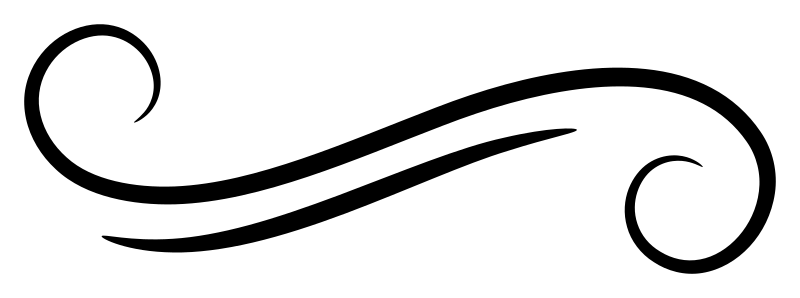Join Type Classes for courses and projects to get you started and make you an expert in FP with Haskell.